Next: Simple Pendulum Up: One-Dimensional Motion Previous: Periodic Driving Forces

Transients

We saw, in Section 3.7, that when a one-dimensional dynamical system, close to a stable equilibrium point, is subject to a sinusoidal external force of the form (104) then the equation of motion of the system is written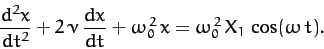(132)

We also found that the solution to this equation which oscillates in sympathy with the applied force takes the form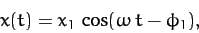(133)

where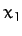and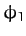are specified in Equations (110) and (111), respectively. However, (133) is not the most general solution to Equation (132). It should be clear that we can take the above solution and add to it any solution of Equation (132) calculated with the right-hand side set to zero, and the result will also be a solution of Equation (132). Now, we investigated the solutions to (132) with the right-hand set to zero in Section 3.5. In the underdamped regime (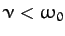), we found that the most general such solution takes the form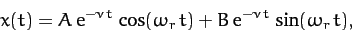(134)

whereandare two arbitrary constants [they are in fact the integration constants of the second-order ordinary differential equation (132)], and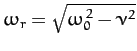. Thus, the most general solution to Equation (132) is written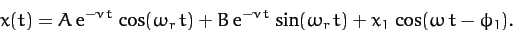(135)

The first two terms on the right-hand side of the above equation are called transients, since they decay in time. The transients are determined by the initial conditions. However, if we wait long enough after setting the system into motion then the transients will always decay away, leaving the time-asymptotic solution (133), which is independent of the initial conditions.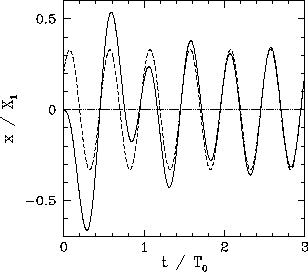As an example, suppose that we set the system into motion at time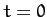with the initial conditions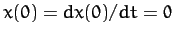. Setting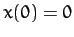in Equation (135), we obtain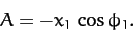(136)

Moreover, setting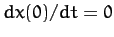in Equation (135), we get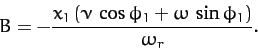(137)

Thus, we have now determined the constantsand, and, hence, fully specified the solution for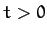. Figure 8 shows this solution (solid curve) calculated for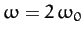and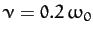. Here,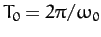. The associated time-asymptotic solution (133) is also shown for the sake of comparison (dashed curve). It can be seen that the full solution quickly converges to the time-asymptotic solution.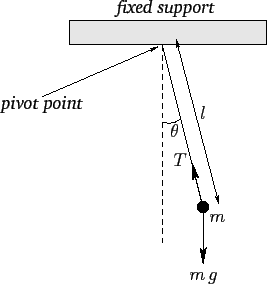Next: Simple Pendulum Up: One-Dimensional Motion Previous: Periodic Driving Forces
Richard Fitzpatrick 2011-03-31